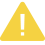# leetcode938(二叉搜索树的范围和)--C语言实现

【摘要】 求：给定二叉搜索树的根结点 root，返回L和R（含）之间的所有结点的值的和。二叉搜索树保证具有唯一的值。 示例1：输入：root=[10,5,15,3,7,null,18],L=7,R=15输出：32示例 2：输入：root=[10,5,15,3,7,13,18,1,null,6],L=6,R=10输出：23 提示：树中的结点数量最多为 10000 个。最终的答案保证小于 2...

/**
* Definition for a binary tree node.
* struct TreeNode {
*     int val;
*     struct TreeNode *left;
*     struct TreeNode *right;
* };
*/

int  rangeSumBST( struct  TreeNode* root,  int  L,  int  R){
if (root==NULL)   return   0 ;
if (root->val < L){
return  rangeSumBST(root->right,L,R);
} else   if (root->val > R){
return  rangeSumBST(root->left,L,R);
} else {
return  root->val + rangeSumBST(root->left,L,R) + rangeSumBST(root->right,L,R);
}
}

/**
* Definition for a binary tree node.
* public class TreeNode {
*     int val;
*     TreeNode left;
*     TreeNode right;
*     TreeNode(int x) { val = x; }
* }
*/
class  Solution {
public   int  rangeSumBST(TreeNode root,  int  L,  int  R) {
int  ret =  0 ;
while (!q.isEmpty()){
TreeNode t = q.poll();
if (t!=null){
if (t.val >=L && t.val <=R){
ret += t.val;
}
if (t.val > L && t.left!=null){
}
if (t.val < R && t.right!=null){
}
}
}
return  ret;
}
}

【版权声明】本文为华为云社区用户转载文章，如果您发现本社区中有涉嫌抄袭的内容，欢迎发送邮件至：huaweicloud.bbs@huawei.com进行举报，并提供相关证据，一经查实，本社区将立刻删除涉嫌侵权内容。

### 评论 (0)

###### 温馨提示